Anúncio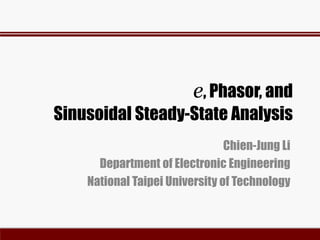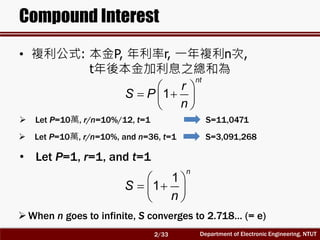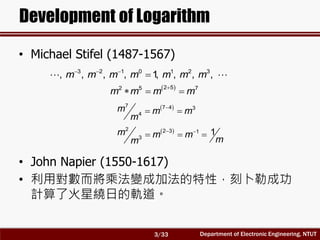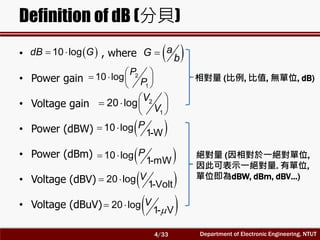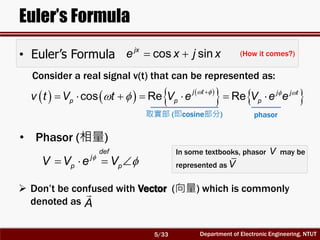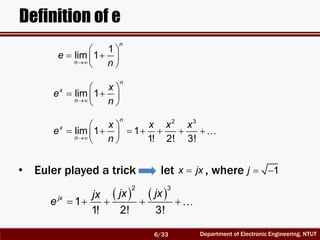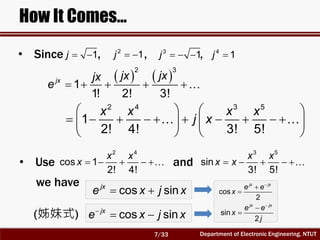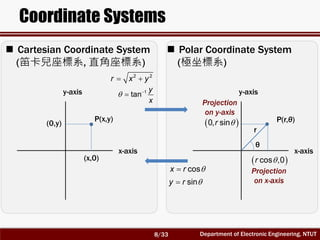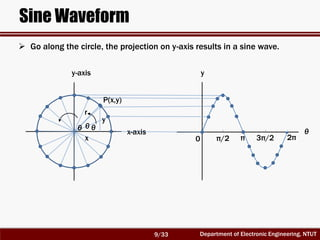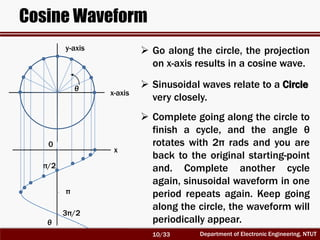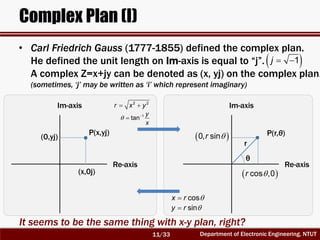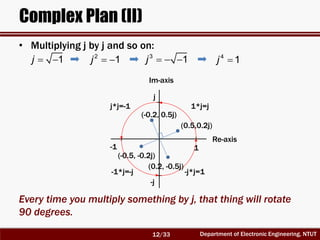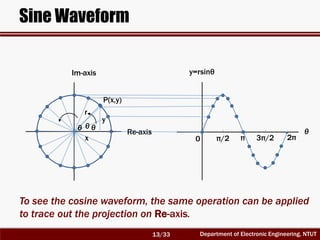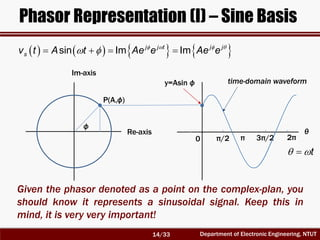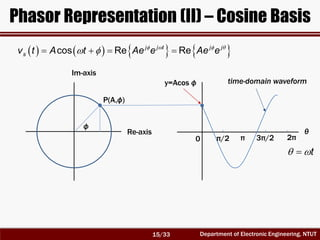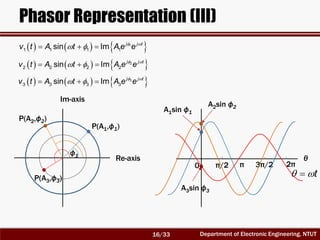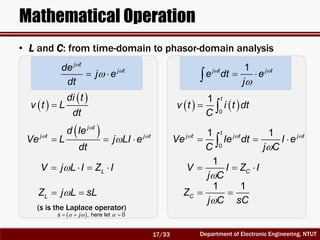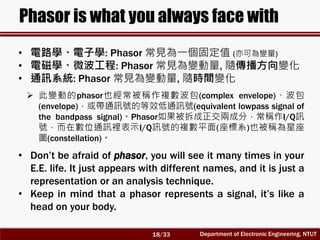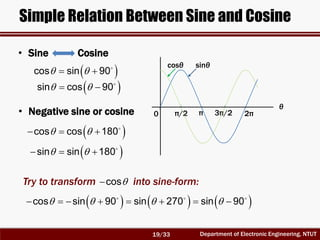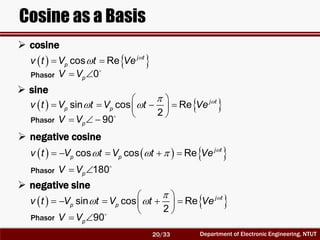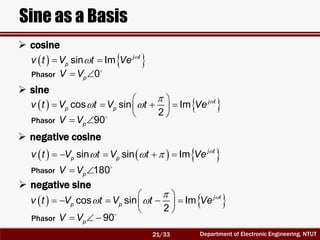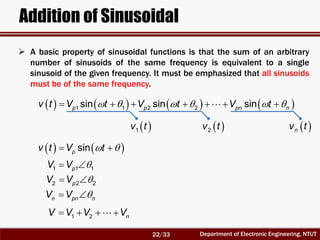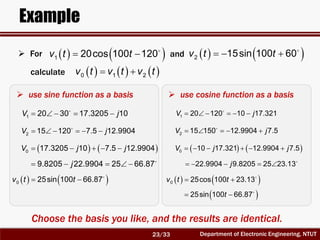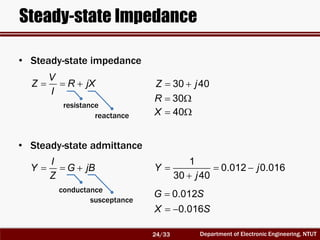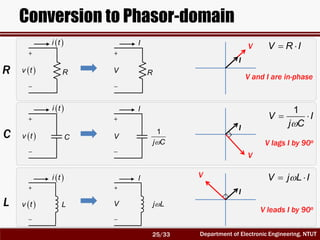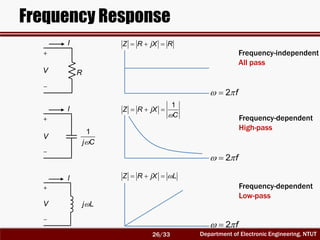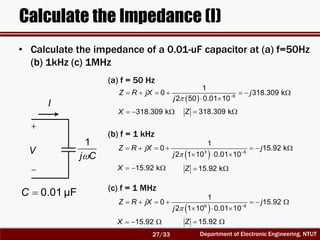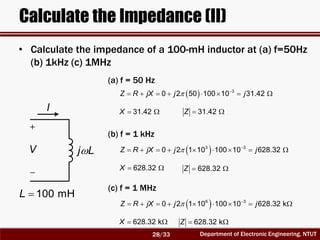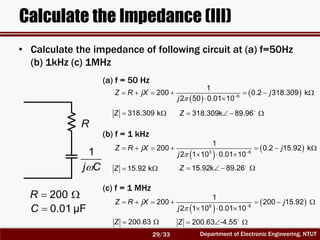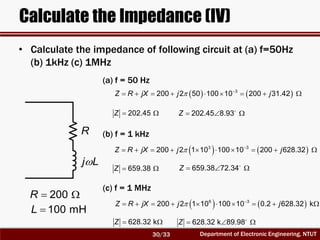1 de 30
Anúncio

### RF Circuit Design - [Ch1-1] Sinusoidal Steady-state Analysis

1. e, Phasor, and Sinusoidal Steady-State Analysis Chien-Jung Li Department of Electronic Engineering National Taipei University of Technology
2. Department of Electronic Engineering, NTUT Compound Interest • 複利公式: 本金P, 年利率r, 一年複利n次, t年後本金加利息之總和為        1 nt r S P n • Let P=1, r=1, and t=1        1 1 n S n When n goes to infinite, S converges to 2.718… (= e)  Let P=10萬, r/n=10%/12, t=1 S=11,0471  Let P=10萬, r/n=10%, and n=36, t=1 S=3,091,268 2/33
3. Department of Electronic Engineering, NTUT Development of Logarithm • Michael Stifel (1487-1567) • John Napier (1550-1617) • 利用對數而將乘法變成加法的特性，刻卜勒成功 計算了火星繞日的軌道。      2 52 5 7 m m m m     7 7 4 3 4 m m m m       2 2 3 1 3 1m m m mm    3 2 1 0 1 2 3 , , , , 1, , , ,m m m m m m m 3/33
4. Department of Electronic Engineering, NTUT Definition of dB (分貝) • , where • Power gain • Voltage gain • Power (dBW) • Power (dBm) • Voltage (dBV) • Voltage (dBuV)   10 logdB G   aG b        2 1 10 log P P        2 1 20 log V V   10 log 1-W P   10 log 1-mW P   20 log 1-Volt V    20 log 1- V V 相對量 (比例, 比值, 無單位, dB) 絕對量 (因相對於一絕對單位, 因此可表示一絕對量. 有單位, 單位即為dBW, dBm, dBV…) 4/33
5. Department of Electronic Engineering, NTUT In some textbooks, phasor may be represented as Euler’s Formula • Euler’s Formula cos sinjx e x j x                        cos Re Re j t j j t p p pv t V t V e V e e      def j p pV V e V • Phasor (相量)  Don’t be confused with Vector (向量) which is commonly denoted as A (How it comes?) 取實部 (即cosine部分) phasor Consider a real signal v(t) that can be represented as: V V 5/33
6. Department of Electronic Engineering, NTUT Definition of e lim 1 n x n x e n        2 3 lim 1 1 1! 2! 3! n x n x x x x e n             x jx     2 3 1 1! 2! 3! jx jx jxjx e      • Euler played a trick let , where 1j   1 lim 1 n n e n        6/33
7. Department of Electronic Engineering, NTUT • Since , , , How It Comes… 1j   2 1j   3 1j    4 1j                       2 4 3 5 1 2! 4! 3! 5! x x x x j x 2 4 cos 1 2! 4! x x x      3 5 sin 3! 5! x x x x     cos sinjx e x j x  cos sinjx e x j x   cos 2 jx jx e e x      sin 2 jx jx e e x j         2 3 1 1! 2! 3! jx jx jxjx e • Use and we have (姊妹式) 7/33
8. Department of Electronic Engineering, NTUT Coordinate Systems x-axis y-axis x-axis y-axis P(r,θ) θ r P(x,y) 2 2 r x y  1 tan y x    cosx r  siny r   Cartesian Coordinate System (笛卡兒座標系, 直角座標系)  Polar Coordinate System (極坐標系) (x,0) (0,y)  cos ,0r   0, sinr  Projection on x-axis Projection on y-axis 8/33
9. Department of Electronic Engineering, NTUT Sine Waveform x-axis y-axis P(x,y) x y r θ θθ y θ 0 π/2 π 3π/2 2π  Go along the circle, the projection on y-axis results in a sine wave. 9/33
10. Department of Electronic Engineering, NTUT x θ 0 π/2 π 3π/2 Cosine Waveform x-axis y-axis θ  Go along the circle, the projection on x-axis results in a cosine wave.  Sinusoidal waves relate to a Circle very closely.  Complete going along the circle to finish a cycle, and the angle θ rotates with 2π rads and you are back to the original starting-point and. Complete another cycle again, sinusoidal waveform in one period repeats again. Keep going along the circle, the waveform will periodically appear. 10/33
11. Department of Electronic Engineering, NTUT Complex Plan (I) It seems to be the same thing with x-y plan, right? • Carl Friedrich Gauss (1777-1855) defined the complex plan. He defined the unit length on Im-axis is equal to “j”. A complex Z=x+jy can be denoted as (x, yj) on the complex plan. (sometimes, ‘j’ may be written as ‘i’ which represent imaginary) Re-axis Im-axis Re-axis Im-axis P(r,θ) θ r P(x,yj) 2 2 r x y  1 tan y x    cosx r  siny r  (x,0j) (0,yj)  cos ,0r   0, sinr   1j   11/33
12. Department of Electronic Engineering, NTUT Complex Plan (II) Re-axis Im-axis 1 Every time you multiply something by j, that thing will rotate 90 degrees. 1j   2 1j   3 1j    4 1j  1*j=j j j*j=-1 -1 -j -1*j=-j -j*j=1 (0.5,0.2j) (-0.2, 0.5j) (-0.5, -0.2j) (0.2, -0.5j) • Multiplying j by j and so on: 12/33
13. Department of Electronic Engineering, NTUT Sine Waveform Re-axis Im-axis P(x,y) x y r θ θθ y=rsinθ θ 0 π/2 π 3π/2 2π To see the cosine waveform, the same operation can be applied to trace out the projection on Re-axis. 13/33
14. Department of Electronic Engineering, NTUT Phasor Representation (I) – Sine Basis                sin Im Imj j t j j sv t A t Ae e Ae e Re-axis Im-axis P(A,ф) y=Asin ф θ 0 π/2 π 3π/2 2π ф t  Given the phasor denoted as a point on the complex-plan, you should know it represents a sinusoidal signal. Keep this in mind, it is very very important! time-domain waveform 14/33
15. Department of Electronic Engineering, NTUT Phasor Representation (II) – Cosine Basis                cos Re Rej j t j j sv t A t Ae e Ae e Re-axis Im-axis P(A,ф) y=Acos ф θ 0 π/2 π 3π/2 2π ф t  time-domain waveform 15/33
16. Department of Electronic Engineering, NTUT Phasor Representation (III)            1 1 1 1 1sin Im j j t v t A t A e e Re-axis Im-axis P(A1,ф1) ф1 P(A2,ф2) P(A3,ф3) θ 0 π/2 π 3π/2 2π t  A1sin ф1            2 2 2 2 2sin Im j j t v t A t A e e            3 3 3 3 3sin Im j j t v t A t A e e A2sin ф2 A3sin ф3 16/33
17. Department of Electronic Engineering, NTUT Mathematical Operation j t j tde j e dt     1j t j t e dt e j         0 1 t v t i t dt C         0 1 1t j t j t j t Ve Ie dt I e C j C     1 CV I Z I j C    di t v t L dt         j t j t j t d Ie Ve L j LI e dt    LV j L I Z I    1 1 CZ j C sC  LZ j L sL • L and C: from time-domain to phasor-domain analysis (s is the Laplace operator)      , here let 0s j 17/33
18. Department of Electronic Engineering, NTUT Phasor is what you always face with • 電路學、電子學: Phasor 常見為一個固定值 (亦可為變量) • 電磁學、微波工程: Phasor 常見為變動量, 隨傳播方向變化 • 通訊系統: Phasor 常見為變動量, 隨時間變化  此變動的phasor也經常被稱作複數波包(complex envelope)、波包 (envelope)，或帶通訊號的等效低通訊號(equivalent lowpass signal of the bandpass signal)。Phasor如果被拆成正交兩成分，常稱作I/Q訊 號，而在數位通訊裡表示I/Q訊號的複數平面(座標系)也被稱為星座 圖(constellation)。 • Don’t be afraid of phasor, you will see it many times in your E.E. life. It just appears with different names, and it is just a representation or an analysis technique. • Keep in mind that a phasor represents a signal, it’s like a head on your body. 18/33
19. Department of Electronic Engineering, NTUT Simple Relation Between Sine and Cosine • Sine Cosine π/2 π 3π/2 2π sinθ θ 0 cosθ • Negative sine or cosine    cos sin 90    sin cos 90     cos cos 180     sin sin 180 Try to transform into sine-form:cos                cos sin 90 sin 270 sin 90 19/33
20. Department of Electronic Engineering, NTUT Cosine as a Basis      cos Re j t pv t V t Ve  0pV V                sin cos Re 2 j t p pv t V t V t Ve    90pV V             cos cos Re j t p pv t V t V t Ve  180pV V                 sin cos Re 2 j t p pv t V t V t Ve  90pV V  cosine  sine  negative cosine  negative sine Phasor Phasor Phasor Phasor 20/33
21. Department of Electronic Engineering, NTUT Sine as a Basis      sin Im j t pv t V t Ve  0pV V                cos sin Im 2 j t p pv t V t V t Ve  90pV V             sin sin Im j t p pv t V t V t Ve  180pV V                 cos sin Im 2 j t p pv t V t V t Ve    90pV V Phasor Phasor Phasor Phasor  cosine  sine  negative cosine  negative sine 21/33
22. Department of Electronic Engineering, NTUT Addition of Sinusoidal  A basic property of sinusoidal functions is that the sum of an arbitrary number of sinusoids of the same frequency is equivalent to a single sinusoid of the given frequency. It must be emphasized that all sinusoids must be of the same frequency.      sinpv t V t  1 1 1pV V  2 2 2pV V  n pn nV V    1 2 nV V V V                   1 1 2 2sin sin sinp p pn nv t V t V t V t  1v t  2v t  nv t 22/33
23. Department of Electronic Engineering, NTUT Example       0 1 2v t v t v t     1 20cos 100 120v t t      2 15sin 100 60v t t     1 20 30 17.3205 10V j      2 15 120 7.5 12.9904V j        0 17.3205 10 7.5 12.9904V j j     0 25sin 100 66.87v t t     9.8205 22.9904 25 66.87j      1 20 120 10 17.321V j     2 15 150 12.9904 7.5V j         0 10 17.321 12.9904 7.5V j j     22.9904 9.8205 25 23.13j     0 25cos 100 23.13v t t   25sin 100 66.87t Choose the basis you like, and the results are identical. and For calculate  use sine function as a basis  use cosine function as a basis 23/33
24. Department of Electronic Engineering, NTUT Steady-state Impedance    V Z R jX I • Steady-state impedance resistance reactance    I Y G jB Z • Steady-state admittance conductance susceptance  30 40Z j  30R  40X     1 0.012 0.016 30 40 Y j j  0.012G S  0.016X S 24/33
25. Department of Electronic Engineering, NTUT Conversion to Phasor-domain  i t  v t V I RR  i t  v t  i t  v t C L  1 j C V I j LV I  V R I    1 V I j C  V j L I V I V I V I V and I are in-phase V lags I by 90o V leads I by 90o R C L 25/33
26. Department of Electronic Engineering, NTUT Frequency Response Frequency-independent All pass Frequency-dependent High-pass Frequency-dependent Low-pass V I R  1 j C V I j LV I   Z R jX R     1 Z R jX C   2 f   2 f   2 f   Z R jX L 26/33
27. Department of Electronic Engineering, NTUT Calculate the Impedance (I)  1 j C V • Calculate the impedance of a 0.01-uF capacitor at (a) f=50Hz (b) 1kHz (c) 1MHz             6 1 0 318.309 k 2 50 0.01 10 Z R jX j j   318.309 kX  318.309 kZ I (a) f = 50 Hz             3 6 1 0 15.92 k 2 1 10 0.01 10 Z R jX j j   15.92 kX  15.92 kZ (b) f = 1 kHz             6 6 1 0 15.92 2 1 10 0.01 10 Z R jX j j   15.92X  15.92Z (c) f = 1 MHz  0.01 μFC 27/33
28. Department of Electronic Engineering, NTUT Calculate the Impedance (II) • Calculate the impedance of a 100-mH inductor at (a) f=50Hz (b) 1kHz (c) 1MHz           3 0 2 50 100 10 31.42Z R jX j j  31.42X  31.42Z (a) f = 50 Hz            3 3 0 2 1 10 100 10 628.32Z R jX j j  628.32X  628.32Z (b) f = 1 kHz            6 3 0 2 1 10 100 10 628.32 kZ R jX j j  628.32 kX  628.32 kZ (c) f = 1 MHz j LV I  100 mHL 28/33
29. Department of Electronic Engineering, NTUT Calculate the Impedance (III) • Calculate the impedance of following circuit at (a) f=50Hz (b) 1kHz (c) 1MHz                6 1 200 0.2 318.309 k 2 50 0.01 10 Z R jX j j  318.309 kZ (a) f = 50 Hz                3 6 1 200 0.2 15.92 k 2 1 10 0.01 10 Z R jX j j  15.92 kZ (b) f = 1 kHz                6 6 1 200 200 15.92 2 1 10 0.01 10 Z R jX j j  200.63Z (c) f = 1 MHz  1 j C  0.01 μFC R  200R    318.309k 89.96Z    15.92k 89.26Z   200.63 -4.55Z 29/33
30. Department of Electronic Engineering, NTUT Calculate the Impedance (IV) • Calculate the impedance of following circuit at (a) f=50Hz (b) 1kHz (c) 1MHz              3 200 2 50 100 10 200 31.42Z R jX j j  202.45Z (a) f = 50 Hz               3 3 200 2 1 10 100 10 200 628.32Z R jX j j  659.38Z (b) f = 1 kHz               6 3 200 2 1 10 100 10 0.2 628.32 kZ R jX j j  628.32 kZ (c) f = 1 MHz j L  100 mHL R  200R   202.45 8.93Z   659.38 72.34Z   628.32 k 89.98Z 30/33
Anúncio